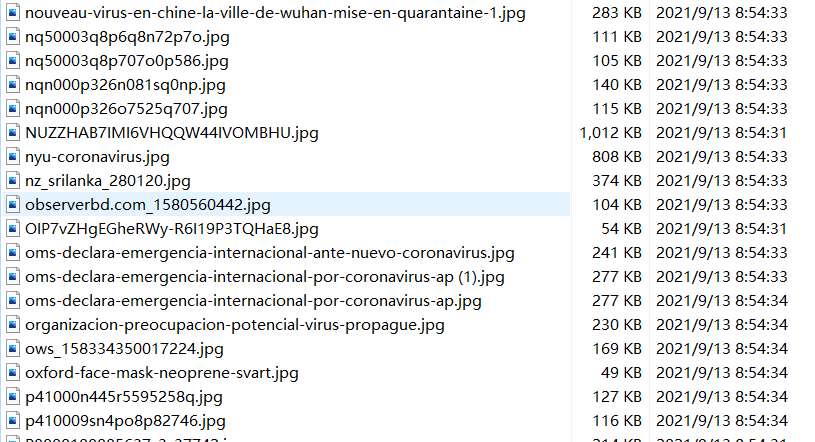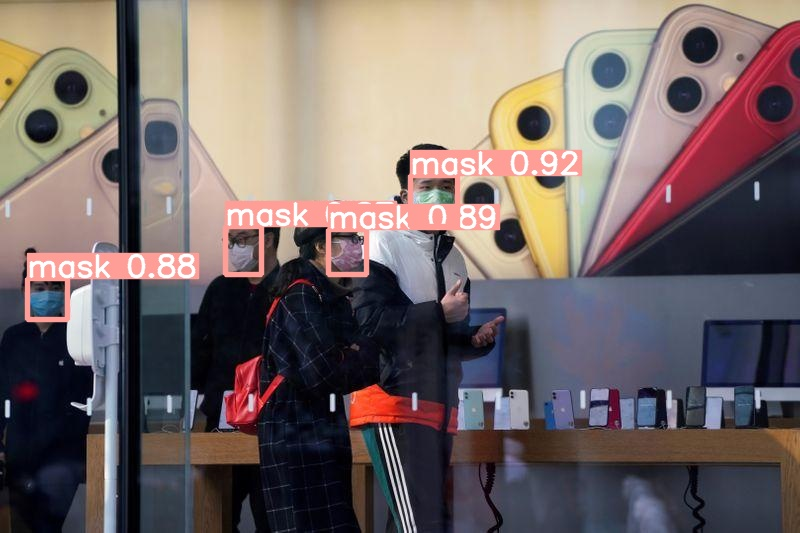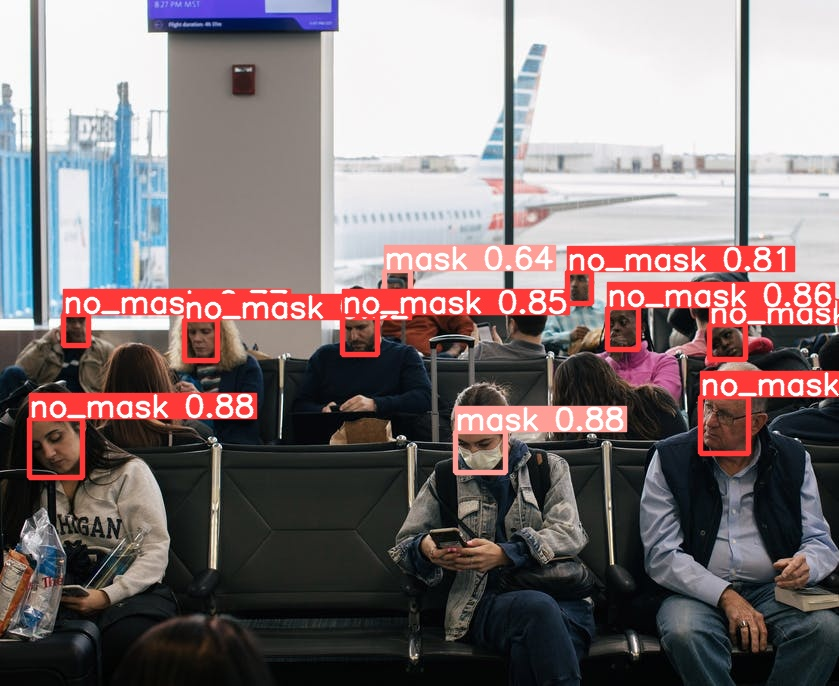一、准备深度学习环境

1、创建数据集

2、转换数据格式

3、配置文件

三、模型训练

1、下载预训练模型

2、训练

五、模型推理

YOLOv5训练自己的数据集整个过程主要包括：环境安装----制作数据集----模型训练----模型测试----模型推理

# 一、准备深度学习环境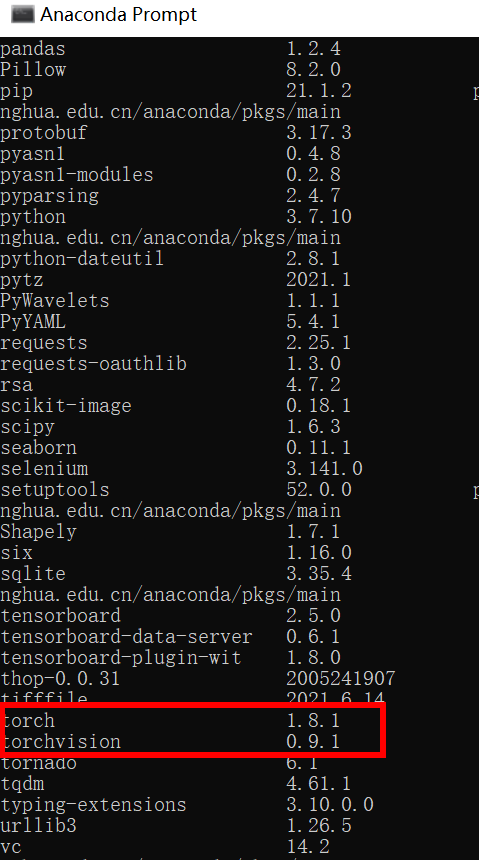# 二、 准备自己的数据集

### 1、创建数据集

mydata
…images # 存放图片
…xml # 存放图片对应的xml文件
…dataSet #之后会在Main文件夹内自动生成train.txt，val.txt，test.txt和trainval.txt四个文件，存放训练集、验证集、测试集图片的名字（无后缀.jpg）

mydata文件夹下内容如下：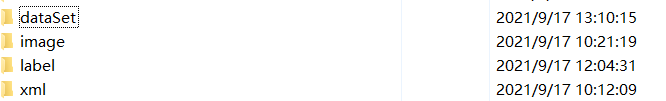• image为VOC数据集格式中的JPEGImages，内容如下：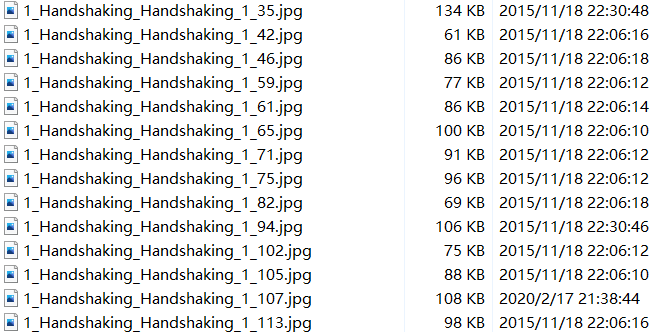• xml文件夹下面为.xml文件（标注工具采用labelImage），内容如下：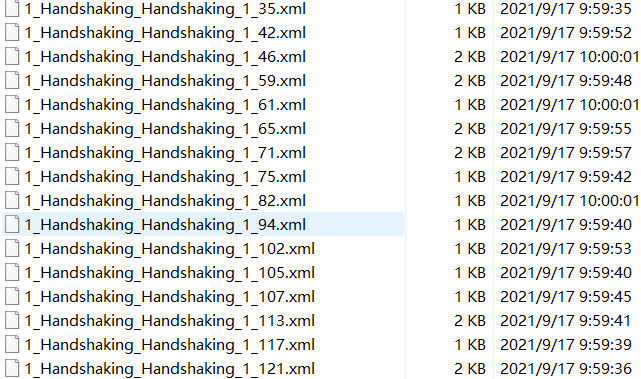• dataSet 文件夹下面存放训练集、验证集、测试集的划分，通过脚本生成，可以创建一个split_train_val.py文件，代码内容如下：
``````# coding:utf-8

import os
import random
import argparse

parser = argparse.ArgumentParser()
# xml文件的地址，根据自己的数据进行修改 xml一般存放在Annotations下
parser.add_argument('--xml_path', default='xml', type=str, help='input xml label path')
# 数据集的划分，地址选择自己数据下的ImageSets/Main
parser.add_argument('--txt_path', default='dataSet', type=str, help='output txt label path')
opt = parser.parse_args()

trainval_percent = 1.0
train_percent = 0.9
xmlfilepath = opt.xml_path
txtsavepath = opt.txt_path
total_xml = os.listdir(xmlfilepath)
if not os.path.exists(txtsavepath):
os.makedirs(txtsavepath)

num = len(total_xml)
list_index = range(num)
tv = int(num * trainval_percent)
tr = int(tv * train_percent)
trainval = random.sample(list_index, tv)
train = random.sample(trainval, tr)

file_trainval = open(txtsavepath + '/trainval.txt', 'w')
file_test = open(txtsavepath + '/test.txt', 'w')
file_train = open(txtsavepath + '/train.txt', 'w')
file_val = open(txtsavepath + '/val.txt', 'w')

for i in list_index:
name = total_xml[i][:-4] + '\n'
if i in trainval:
file_trainval.write(name)
if i in train:
file_train.write(name)
else:
file_val.write(name)
else:
file_test.write(name)

file_trainval.close()
file_train.close()
file_val.close()
file_test.close()
``````
•  运行代码后，在dataSet 文件夹下生成下面四个txt文档：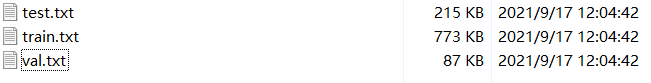• 三个txt文件里面的内容如下：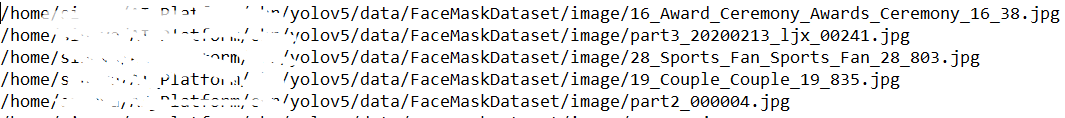### 2、转换数据格式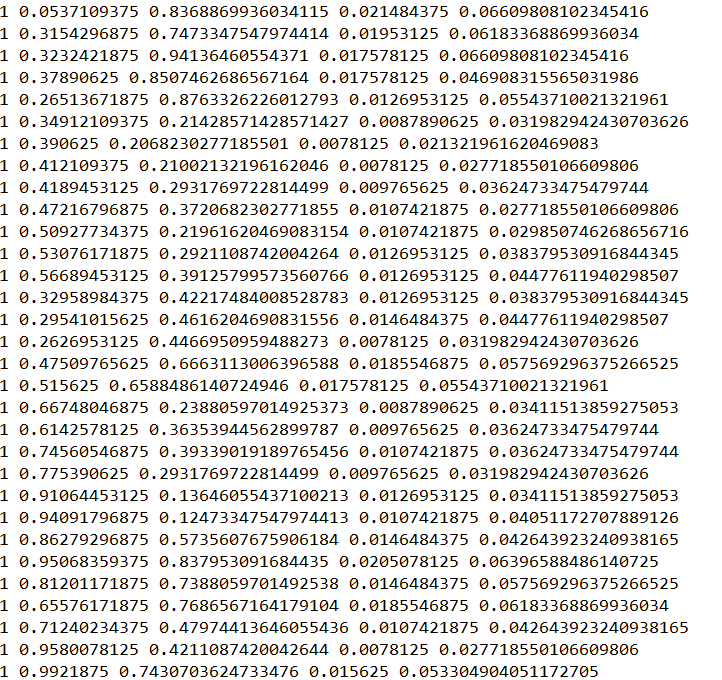•  创建voc_label.py文件，将训练集、验证集、测试集生成label标签（训练中要用到），同时将数据集路径导入txt文件中，代码内容如下：
``````# -*- coding: utf-8 -*-
import xml.etree.ElementTree as ET
import os
from os import getcwd

sets = ['train', 'val', 'test']
classes = ["a", "b"]   # 改成自己的类别
abs_path = os.getcwd()
print(abs_path)

def convert(size, box):
dw = 1. / (size)
dh = 1. / (size)
x = (box + box) / 2.0 - 1
y = (box + box) / 2.0 - 1
w = box - box
h = box - box
x = x * dw
w = w * dw
y = y * dh
h = h * dh
return x, y, w, h

def convert_annotation(image_id):
in_file = open('data/mydata/xml/%s.xml' % (image_id), encoding='UTF-8')
out_file = open('data/mydata/labels/%s.txt' % (image_id), 'w')
tree = ET.parse(in_file)
root = tree.getroot()
size = root.find('size')
w = int(size.find('width').text)
h = int(size.find('height').text)
for obj in root.iter('object'):
# difficult = obj.find('difficult').text
difficult = obj.find('Difficult').text
cls = obj.find('name').text
if cls not in classes or int(difficult) == 1:
continue
cls_id = classes.index(cls)
xmlbox = obj.find('bndbox')
b = (float(xmlbox.find('xmin').text), float(xmlbox.find('xmax').text), float(xmlbox.find('ymin').text),
float(xmlbox.find('ymax').text))
b1, b2, b3, b4 = b
# 标注越界修正
if b2 > w:
b2 = w
if b4 > h:
b4 = h
b = (b1, b2, b3, b4)
bb = convert((w, h), b)
out_file.write(str(cls_id) + " " + " ".join([str(a) for a in bb]) + '\n')

wd = getcwd()
for image_set in sets:
if not os.path.exists('data/mydata/labels/'):
os.makedirs('data/mydata/labels/')
list_file = open('paper_data/%s.txt' % (image_set), 'w')
for image_id in image_ids:
list_file.write(abs_path + '/mydata/images/%s.jpg\n' % (image_id))
convert_annotation(image_id)
list_file.close()
``````

### 3、配置文件

1）数据集的配置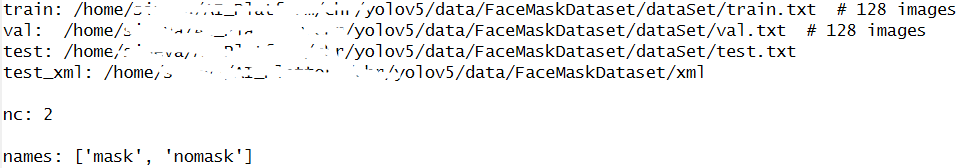2) 选择一个你需要的模型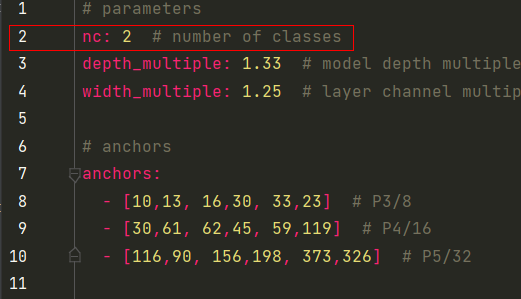至此，自定义数据集已创建完毕，接下来就是训练模型了。

# 三、模型训练

### 1、下载预训练模型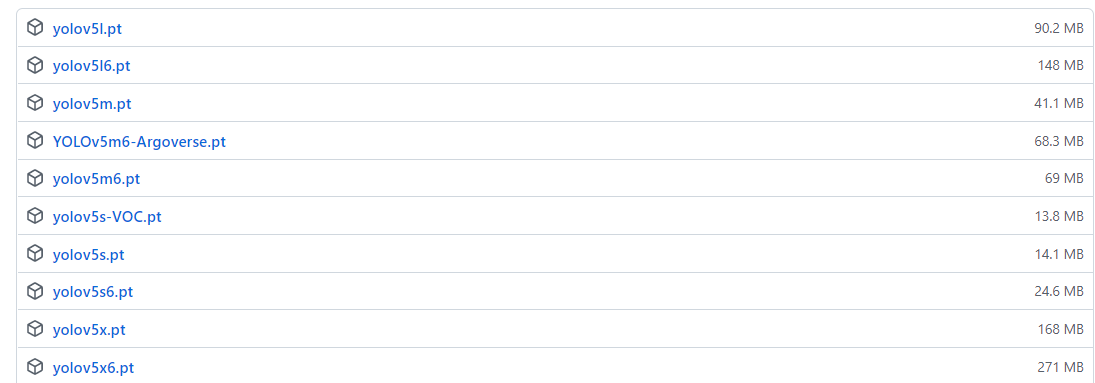### 2、训练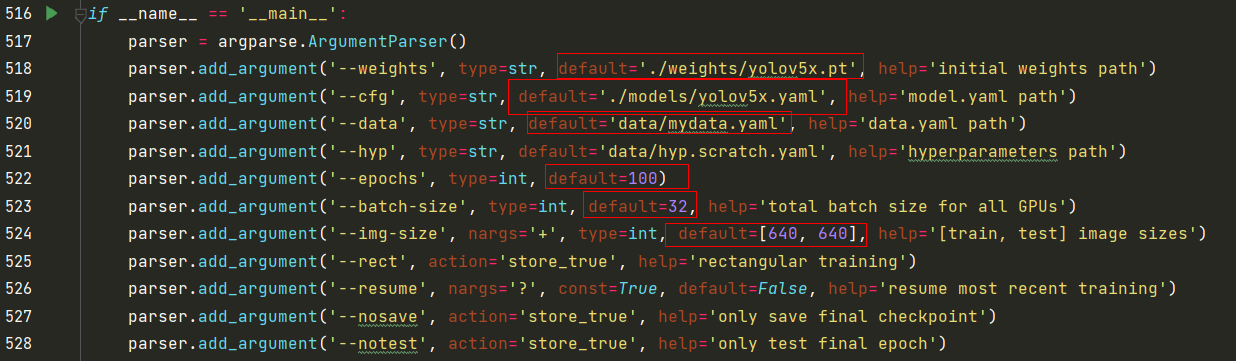epochs：指的就是训练过程中整个数据集将被迭代多少次,显卡不行你就调小点。
batch-size：一次看完多少张图片才进行权重更新，梯度下降的mini-batch,显卡不行你就调小点。
cfg：存储模型结构的配置文件
data：存储训练、测试数据的文件
img-size：输入图片宽高,显卡不行你就调小点。

``python train.py --img 640 --batch 32 --epoch 300 --data data/mydata.yaml --cfg models/yolov5x.yaml --weights weights/yolov5x.pt --device '0,1' ``

# 四、模型测试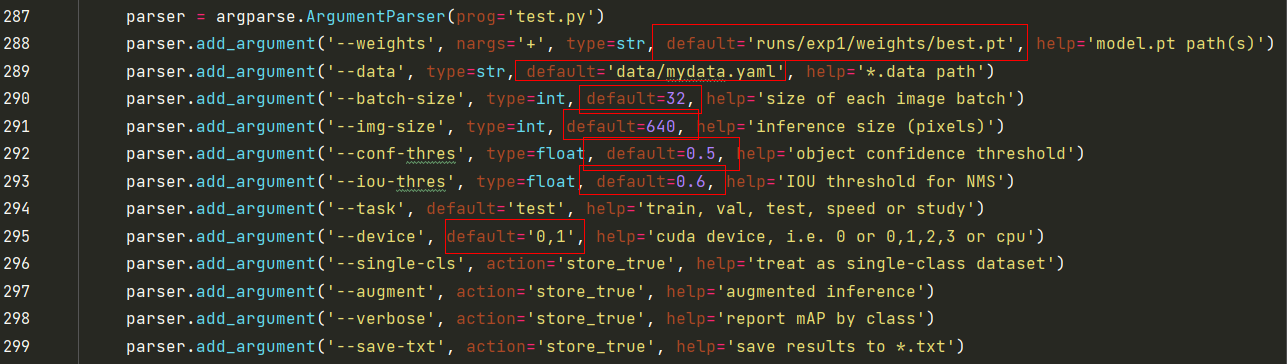``````python test.py  --data data/mydata.yaml --weights runs/exp1/weights/best.pt --augment
``````

模型测试效果如下：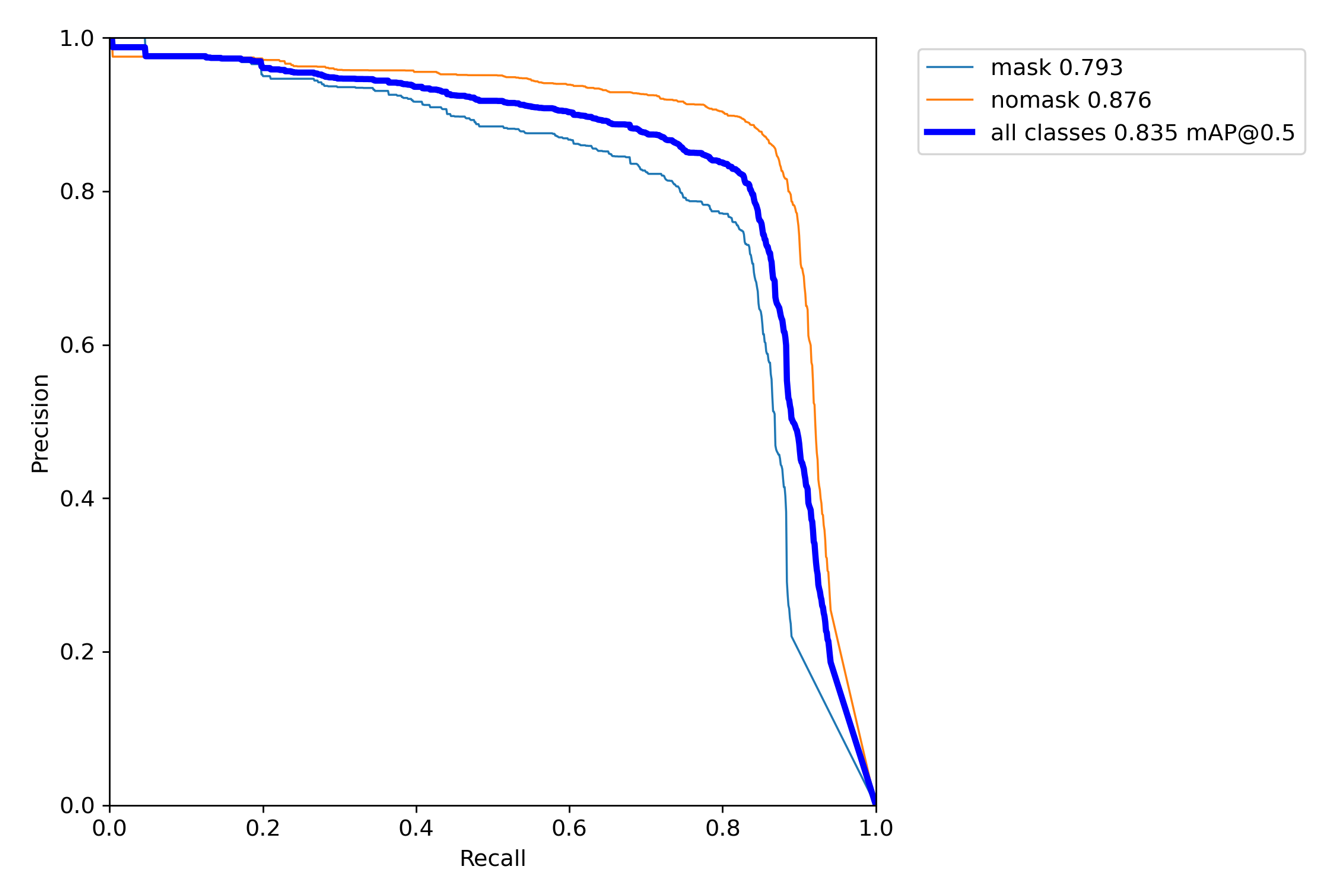# 五、模型推理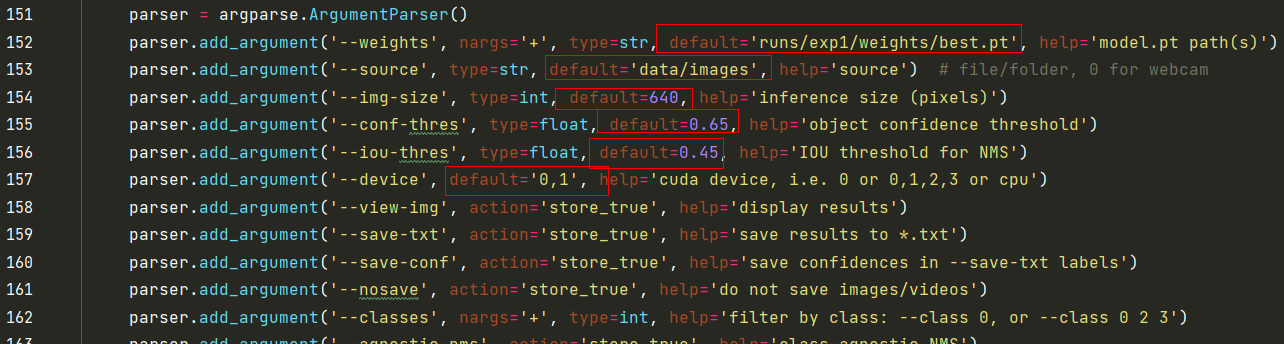`` python detect.py --weights runs/exp1/weights/best.pt --source inference/images/ --device 0,1``